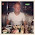## Tuesday, April 26, 2011

### Generating better random numbers with dynamics ax 2009

Inspired by this post, I created a neat little way to generate good random numbers between a range.

If you use AX Random class or RandomGenerate class to generate random numbers, you will find that if you do several in a row, they turn out fairly sequential.

The xGlobal::randomPositiveInt32() does a slightly better job of producing a random integer.

I would sometimes see patterns using this when the output is sorted:

```static void Job6(Args _args)
{
int total = 20;
int i;
;

while (total)
{
i = xGlobal::randomPositiveInt32();

/*
// This is better
while (i > 9999999)
i = i div 10;

while (i < 1000000)
i = i * 10;
*/

while (i > 9999999)
i = i >> 1;

while (i < 1000000)
i = i << 1;

info(int2str(i));

total--;

}
}```

1.Hi, bitwise shifts that you are doing break uniform distribution of the numbers. The simpliest way to get close to uniform distribution on the range [1000000, 9999999) is the following:

xGlobal::randomPositiveInt32() mod (9999999 - 1000000) + 1000000

2.@Gigz, I thought the whole point of uniform distribution was an equal chance of getting any number on a range. It seemed like the results I get, no matter what seem to follow a pattern. Try this job and sort the table. It seems like it'll always start at the low end, and evenly move up until it's at the high end.

static void TASGenerateRandomNumbers(Args _args)
{
#define.totalToGenerate (10000)
#define.high (9999999)
#define.low (1000000)

TASRandomNumbers tas;
int totalToGenerate = #totalToGenerate;
int64 totalOps;
int i;
;

ttsbegin;

delete_from tas;

while (totalToGenerate)
{
// Uncomment this
i = xGlobal::randomPositiveInt32(); //mod (#high - #low) + #low;

// Comment this out
while (i > #high)
i = i div 10;

// Comment this out
while (i < #low)
i = i * 10;

if (TASRandomNumbers::exist(i) == NoYes::No)
{
tas.clear();
tas.RandNum = i;
tas.insert();

totalToGenerate--;
}

totalOps++;
}

ttscommit;

info("Total operations: " + int642str(totalOps));
}

3.Yep, you are right about the definition of the uniform distribution.

But to measure if numbers are generated uniformly or not, you need to calculate the frequency of each number (how many times each number is generated).

something like:
(without if (TASRandomNumbers::exist(i) == NoYes::No))

select forupdate tas where tas.RandNum == i;
tas.Freq++;
tas.write()

and then look if all records have pretty much the same Freq values.

This should be done for large number of experiments, at least 100 times larger than interval size.